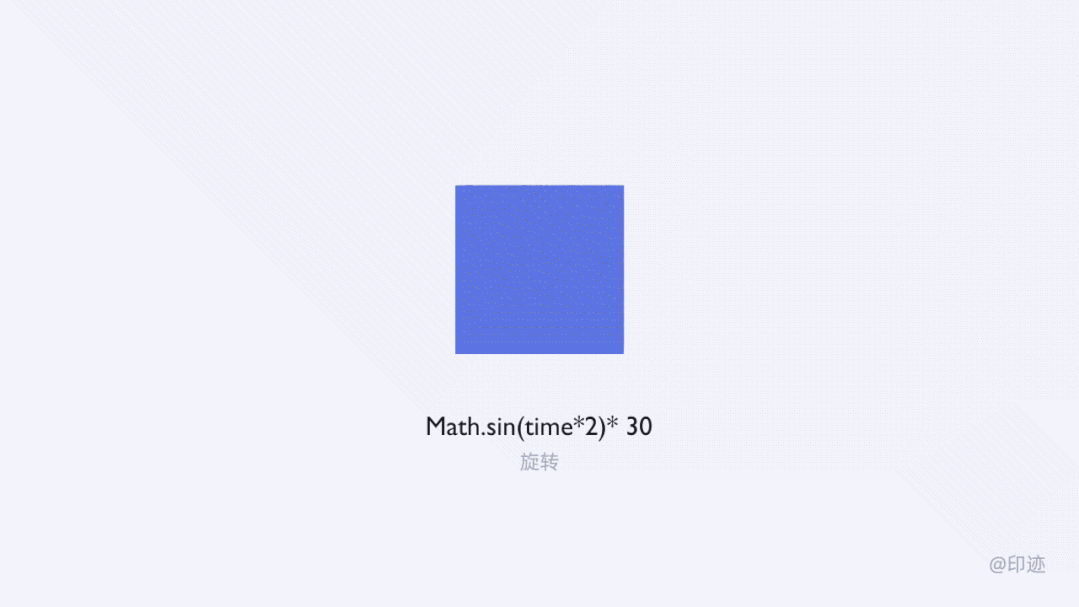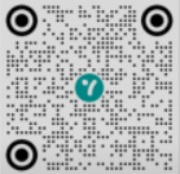# 快速出效果！10条有趣又实用的AE表达式#### time

value 是数值，用在元素的不透明度上，就是由透明到不透明的变化。#### 正弦函数 sin

value1=频率值；value2=幅度值`例如把这行代码 Math.sin(time*2)* 30 加在旋转属性上，就是以 30°的角度来回旋转 2 次,可以代替手动 K 帧的繁琐。``Math.sin(time* value1)* value2/Math.exp(time* value)`

#### wiggle 抖动

value1 为每秒抖动的次数，value2 为每次随机波动的幅度。`wiggle(freq, amp, octaves = 1, amp_mult = 0.5, t = time)`

freq=频率（设置每秒抖动的频率）；amp=振幅（每次抖动的幅度）；octaves=振幅幅度（在每次振幅的基础上还会进行一定的震幅幅度，很少用）；amp_mult=频率倍频（默认数值即可，数值越接近 0，细节越少；越接近 1，细节越多）；t=持续时间（抖动时间为合成时间，一般无需修改）；一般只写前两个数值即可。

#### index#### time 计时#### random 随机

min 是最小值，max 最大值`X=random（1,100）`
`Math.round(X)`“X”是你自定义的名字，意思就是给这个“X”规定，让它只能在 1-100 之间整数变化。这个效果可用在抽奖游戏里的随机数字。

#### 运动脱尾

```delay = 5; //number of frames to delay（延迟的距离）
d = delay*thisComp.frameDuration*(index - 1);
thisComp.layer(1).position.valueAtTime(time - d)``````opacityFactor = .75;//不透明度值
Math.pow(opacityFactor,index - 1)*100```

#### 万能弹性表达式

Keyframe Overshoot

```amp = .1;
freq = 2.0;
decay = 2.0;
n = 0;
if (numKeys > 0){
n = nearestKey(time).index;
if (key(n).time > time){n--;}
}
if (n == 0){ t = 0;}
else{t = time - key(n).time;}
if (n > 0){
v = velocityAtTime(key(n).time - thisComp.frameDuration/10);
value + v*amp*Math.sin(freq*t*2*Math.PI)/Math.exp(decay*t);
}
else{value}```

*amp 表示振幅,freq 表示频率,decay 表示衰减（根据不同需求做不同的调整）Keyframe Bounce Back

```e = .7;
g = 5000;
nMax = 9;
n = 0;
if (numKeys > 0){

n = nearestKey(time).index;
if (key(n).time > time) n--;
}
if (n > 0){
t = time - key(n).time;
v = -velocityAtTime(key(n).time - .001)*e;
vl = length(v);
if (value instanceof Array){
vu = (vl > 0) ? normalize(v) : [0,0,0];
}else{
vu = (v < 0) ? -1 : 1;
}
tCur = 0;
segDur = 2*vl/g;
tNext = segDur;
nb = 1; // number of bounces
while (tNext < t && nb <= nMax){
vl *= e;
segDur *= e;
tCur = tNext;
tNext += segDur;
nb++
}
if(nb <= nMax){
delta = t - tCur;
value + vu*delta*(vl - g*delta/2);
}else{
value
}
}else value```

*e 表示衰减，g 表示重力，nMax 表示弹跳最大值（根据不同需求做不同的调整）

#### loopOut 循环

loopOut(type=“类型”,numkeyframes=0)：对一组动作进行循环 loopOut(type=”pingpong”,numkeyframes=0)：是类似像乒乓球一样的来回循环； loopOut(type=”cycle”,numkeyframes=0)：是周而复始的循环； loopOut(type=”continue”)：延续属性变化的最后速度， loopOut(type=”offset”,numkeyframes=0)：是重复指定的时间段进行循环； numkeyframes=0 是循环的次数，0 为无限循环，1 是最后两个关键帧无限循环，2 是最后三个关键帧无限循环loopOutduration 和 loopInduration

duration = 0 时候一切遵循以上 Out 和 In 的基本解释。

duration = 1，这里分为两种：

duration = 1 表示循环该套动作的前 1 秒内(绿色框内 2s-3s)的动作。duration = 1 表示循环该套动作的最后 1 秒内(绿色框内 3s-4s)的动作。#### linear 线性函数

`代码：linear(t,tMin,tMax,value1,value2)`

t=某属性（位置、缩放、不透明度…）线性变化值（tmin 最小，tmax 最大）

value1,value2 代表给当前添加表达式的参数的一维变化。t=(拖动螺旋线指向你想要绑定的那个图层的某个属性，比如 x/y 某一个方向的值)

```t=(你想要绑定的那个图层的某个属性)
kuan=linear(t,tMin,tMax,value1,value2);
gao=linear(t,tMin,tMax,value1,value2);
[kuan,gao]```

## 超实用！18种常用AE表达式解析Author：印迹